Courses

# Sample Question Paper (2020-21) - 3 Class 9 Notes | EduRev

## Class 9 : Sample Question Paper (2020-21) - 3 Class 9 Notes | EduRev

The document Sample Question Paper (2020-21) - 3 Class 9 Notes | EduRev is a part of the Class 9 Course Sample Papers For Class 9.
All you need of Class 9 at this link: Class 9

Class IX
Science
Sample Question Paper 2020-21
Max. Marks : 80
Duration : 3 hrs.

General Instructions:
Read the following instructions very carefully and strictly follow them :
1. The question paper comprises four sections A, B, C and D. There are 36 questions in the question paper. All questions are compulsory.
2. Section–A – question no. 1 to 20 - all questions and parts thereof are of one mark each. These questions contain multiple choice questions (MCQs), very short answer questions and assertion - reason type questions. Answers to these questions should be given in one word or one sentence.
3. Section–B – question no. 21 to 26 are short answer type questions, carrying 2 marks each. Answers to these questions should be in the range of 30 to 50 words.
4. Section–C – question no. 27 to 33 are short answer type questions, carrying 3 marks each. Answers to these questions should be in the range of 50 to 80 words.
5. Section–D – question no. 34 to 36 are long answer type questions carrying 5 marks each. Answer to these questions should be in the range of 80 to 120 words.
6. There is no overall choice. However, internal choices have been provided in some questions. A student has to attempt only one of the alternatives in such questions.
7. Wherever necessary, neat and properly labelled diagrams should be drawn.

Section - A

Q.1. Define 1 kWh.  (1 Mark)
Ans. One kilowatt hour is the amount of electrical energy consumed when an electrical appliance of 1000 watt is used for 1 hour.

OR
Which type of object is supposed to possess energy?  (1 Mark)
Ans. An object having a capacity to do work is said to possess energy.

Q.2. If the mass of a body is doubled. How does its acceleration change under a given force?  (1 Mark)
Ans.
Acceleration becomes half
Detailed Answer:
F = ma
If mass is doubled then, F / 2m = a
or 1 / 2(F / m) = a
Hence, acceleration becomes half.

Q.3. Which of the following figure represents uniform motion of a moving object correctly?  (1 Mark)
(a)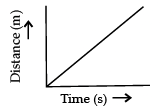(b)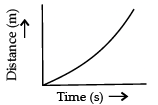(c)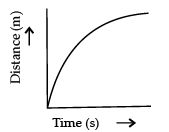(d)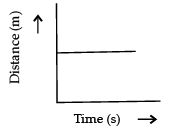Ans. a
Solution. For uniform motion, the distance-time graph is a straight line. This is because in uniform motion object covers equal distance in equal interval of time.

Q.4. Can the displacement be greater than the distance travelled by an object?  (1 Mark)
Ans. No, it is always either equal to or less than the distance travelled by the object.

Q.5. Why is the motion of a circulating fan non-uniform?  (1 Mark)
Ans. The motion of a circulating fan is non-uniform because the direction of motion changes at every point.

Q.6. Which force causes the things to fall towards the earth?   (1 Mark)
OR
When does an object show weightlessness?  (1 Mark)

Ans. Force due to gravity.
OR
Weightlessness is a state when an object does not weigh anything. It occurs only when a body is in a state of free fall under the effect of only gravity.

Q.7. Name the plastid involved in conversion of a green brinjal to violet.  (1 Mark)
Ans.
Chromoplast.

Q.8. What is the measure of angle between the force and displacement if the work done is negative?  (1 Mark)
Ans. In case of negative work, the angle between the force and displacement of 180°.

Q.9. Name two types of mixtures.  (1 Mark)
Ans.
Homogeneous mixtures and heterogeneous mixtures.

OR
What are the constituents of milk?  (1 Mark)
Ans.
Milk is a mixture of water, fat and proteins.

Q.10. What is the primary cause of marasmus?  (1 Mark)
Ans. Primary cause of marasmus is protein deficiency.

Q.11. When a carpet is beaten with a stick it releases dust. Explain why?  (1 Mark)
Ans. As dust possess inertia of rest, it resists the change and falls down.

OR
Name the physical quantity which is measured by rate of change of momentum.  (1 Mark)
Ans. Force

Q.12. Name an organism sensitive to SO2 in air.  (1 Mark)
Ans. Lichens.

OR
State the role of the atmosphere in climate control.  (1 Mark)
Ans.

(i) It prevents sudden increase in temperature during the day light hours.
(ii) It slows down the escape of heat into outer space during the night.

Q.13. Define the atomic mass unit.  (1 Mark)
Ans.
Atomic mass unit is equal to 1/12th the mass of one atom of carbon-12.

For question numbers 14, 15 and 16, two statements are given- one labelled Assertion (A) and the other labelled Reason (R). Select the correct answer to these questions from the codes (a), (b), (c) and (d) as given below :
Assertion: Atom is electrically neutral.
Reason: Equal number of protons and electrons are present in an atom.  (1 Mark)
(a) Both A and R are true, and R is correct explanation of the assertion.
(b) Both A and R are true, but R is not the correct explanation of the assertion.
(c) A is true, but R is false.
(d) A is false, but R is true.
Ans. a
Solution. Atom is electrically neutral because the total positive charge i.e. protons is cancelled by the total negative charge i.e. electrons.

Q.15. For question numbers 15, two statements are given- one labelled Assertion (A) and the other labelled Reason (R). Select the correct answer to these questions from the codes (a), (b), (c) and (d) as given below:
Assertion: Velocity is the speed of an object in a particular direction.
Reason: SI unit of velocity is same as speed.  (1 Mark)
(a) Both A and R are true, and R is correct explanation of the assertion.
(b) Both A and R are true, but R is not the correct explanation of the assertion.
(c) A is true, but R is false.
(d) A is false, but R is true.
Ans. b
Solution. Velocity is the speed of an object moving in a direction, or the displacement of an object in unit time. Speed, on the other hand, is the distance travelled by an object in a given time. SI unit of velocity is same as speed i.e., m/s.

OR
Assertion:
A boy riding on bicycle in a crowded street exhibit non uniform motion.
Reason: The boy covers equal distance in equal interval of time.  (1 Mark)
(a) Both A and R are true, and R is correct explanation of the assertion.
(b) Both A and R are true, but R is not the correct explanation of the assertion.
(c) A is true, but R is false.
(d) A is false, but R is true.
Ans. c
Solution. Boy riding on the bicycle exhibit non uniform motion. He covers unequal distance at different interval of time.

Q.16. Assertion: Chloroplast performs photosynthesis.
Reason: Chloroplast comprises photosynthetic pigments.  (1 Mark)
Ans. a
Solution. Chloroplast is involved in the process of photosynthesis. It contains a photosynthetic pigment called chlorophyll, which traps light energy from the sun which then combines with carbon dioxide and water to convert into chemical energy by forming sugar.

Q. No 17 - 20 contain five sub-parts each. You are expected to answer any four sub parts in these questions.

Q.17. Read the following and answer any four questions from 17 (i) to 17 (v)
The teacher instructed three students A, B and C respectively to prepare a 50 % (mass by volume) solution of sodium hydroxide. ‘A’ dissolved 50 g of NaOH in 100 mL of water, ‘B’ dissolved 50 g of NaOH in 100 g of water while ‘C’ dissolved 50 g of NaOH in water to make 100 mL of solution .

(i) Which one of the following has made the desired solution?  (1 Mark)
(a)
A
(b) B
(c) C
(d) All of them have made desired solution.
Ans. c
Solution. Student C has made desired solution by dissolving 50 g NaOH in water to make the volume of the solution 100 mL.

(ii) Define concentration of a solution.   (1 Mark)
(a)
It indicates how thick the solution is.
(b) It indicates the exact amount of solute dissolved in an exact amount of solvent or solution.
(c) It indicates the volume of solution.
(d) It indicates the strength of solution.
Ans. b

(iii) How will you prepare a 10% solution of sugar?   (1 Mark)
(a)
Dissolve 90 g of sugar in 10 g of water.
(b) Dissolve 10 g of sugar in 10 g of water.
(c) Dissolve 100 g of sugar in 100 g of water.
(d) Dissolve 10 g of sugar in 90 g of water.
Ans. d
Solution. Dissolve 10 g of sugar in (100 – 10) = 90 g of water.

(iv) The two components of a solution are ________ and ________.  (1 Mark)
(a)
The two components of a solution are solute and solvent.
(b) The two components of a solution are mixture and solvent.
(c) The two components of a solution are compound and mixture.
(d) The two components of a solution are solute and water always.
Ans. (a)

(v) Name the solute and solvent in the above solution.   (1 Mark)
(a)
Sodium hydroxide solute and water solvent
(b) Water solute and sodium hydroxide solvent
(c) Sodium hydroxide and water both are solvents, no solute
(d) Sodium hydroxide and water both are solutes, no solute
Ans. (a)

Q.18. Read the following and answer any four questions from 18 (i) to 18 (v).
Manish and Nitish  are provided with mixture of camphor, common salt and soil by their teacher. The teacher has asked them to use the techniques of sublimation, filtration and evaporation to separate this mixture.

(i) Which technique can be used to separate camphor from the mixture easily?   (1 Mark)
(a) Filtration
(b) Sublimation
(c) Evaporation
(d) All of the above at once
Ans. b

(ii) Filtration technique can be used for separating ………. easily.  (1 Mark)
(a)
Camphor and soil
(b) Common salt and soil
(c) Camphor and common salt
(d) Camphor, common salt, soil
Ans. c

(iii) Which technique can be used to separate common salt from water?  (1 Mark)
(a)
Sublimation
(b) Filtration
(c) Evaporation
(d) All of the above at once
Ans. c

(iv) Which technique can be used to separate salt from sea water?  (1 Mark)
(a) Filtration
(b) Sublimation
(c) Distillation
(d) Crystallisation
Ans. d

(v) Name one more substance which can be separated from a mixture by the process of sublimation   (1 Mark)
(a)
Common salt
(b) Alum
(c) Ammonium chloride
(d) Soil
Ans. c

Q.19. Read the following and answer any four questions from 19 (i) to 19 (v)
Two beakers A and B contain plain water and concentrated sugar solution respectively. Equal number of dry raisins are kept in them for a few hours and then taken out.

(i) In beaker A, the raisins would ________ while in beaker B, raisins will ________. (1 Mark)
(a) In beaker A, the raisins would shrink while in beaker B, raisins will swell.
(b) In beaker A, the raisins would swell while in beaker B, raisins will shrink.
(c) In both cases, the raisins would swell.
(d) In both cases, the resins would shrink.
Ans. b

(ii) Give explanation for above observation.  (1 Mark)
(a)
In beaker A, the raisins would shrink because water concentration is higher outside the cell membrane, so more water enters the membrane than leaves it. In beaker B, raisins will swell because water concentration is less outside the cell membrane, so more water comes out of the membrane than enters.
(b) In beaker A, the raisins would swell because water concentration is higher outside the cell membrane, so more water enters the membrane than leaves it. In beaker B, raisins will shrink because water concentration is less outside the cell membrane, so more water comes out of the membrane than enters.
(c) In beaker A, the raisins would swell because water concentration is higher outside the cell membrane, so more water leaves the membrane than enters in it. In beaker B, raisins will shrink because water concentration is more outside the cell membrane, so more water comes out of the membrane than enters.
(d) In beaker A, the raisins would swell because water concentration is higher outside the cell membrane, so more water enters the membrane than leaves it. In beaker B, raisins will shrink because water concentration is less outside the cell membrane, so more water comes out of the membrane than enters.
Ans. b
Solution. In beaker A, the raisins would swell because water concentration is higher outside the cell membrane, so more water enters the membrane than it leaves it. In beaker B, raisins will shrink because water concentration is less outside the cell membrane, so more water comes out of the membrane than it enters.

(iii) On the basis of above observation, categorise the two solutions in the beakers as hypotonic and hypertonic.  (1 Mark)
(a)
In both cases, solutions are hypotonic
(b) In both cases, solutions are hypertonic
(c) Beaker A hypotonic, Beaker B hypertonic
(d) Beaker A hypertonic, Beaker B hypotonic
Ans. c

(iv) Plasmolysis is :   (1 Mark)
(a)
The process of water flowing in and out of cells at an equal rate in an isotonic solution
(b) The process of water flowing out of cells in a hypotonic solution
(c) The process of water flowing in cells in a hypotonic solution
(d) The process of water flowing out of cells in a hypertonic solution
Ans. d

(v) Cells kept in which type of solution do not lose or gain water?  (1 Mark)
(a)
Hypotonic solution
(b) Isotonic solution
(c) Hypertonic solution
(d) Saturated solution
Ans. b

Q.20. The graph shows the distance-time graph of three objects A, B and C. Study the graph and answers the following questions:

(i) Which of the three is travelling the fastest?  (1 Mark)
(a) C
(b) A
(c) B
(d) All are at equal speed
Ans. c
Solution. Since B, it travels the most distance, in the smallest time, it is travelling the fastest.

(ii) Are all three ever at the same point on the road?  (1 Mark)
(a)
Yes
(b) No
Ans. b
Solution. All the three objects A, B and C never meet at a single point. Thus, all the three were never at the same point on the road.

(iii) How far has B travelled in 1.4 hours?  (1 Mark)
(a)
B travelled from 5 to 12 km in 1.4 hours.
(b) B travelled from 1 to 12 km in 1.4 hours.
(c) B travelled from 4 to 12 km in 1.4 hours.
(d) B travelled from 0 to 12 km in 1.4 hours.
Ans. d

(iv) How far has C travelled in 1.6 hours?  (1 Mark)
(a)
C travelled from 8 to 12 km in 1.6 hours.
(b) C travelled from 2 to 12 km in 1.6 hours.
(c) C travelled from 0 to 12 km in 1.6 hours.
(d) C travelled from 1 to 12 km in 1.6 hours.
Ans. b

(v) State the formula used to find out speed from distance time graph.  (1 Mark)
(a)
Speed = Time / Distance
(b) Speed = Distance × Time
(c) Speed = Distance/Time
(d) Time = Speed × Distance
Ans. c

Section - B

Q.21. Write two similarities and one dissimilarity between mitochondria and plastid.  (2 Mark)
OR
State the differences between tendon and ligament. (2 Mark)
Ans. Both are double membrane structures. Both of them have their own genetic material.
OR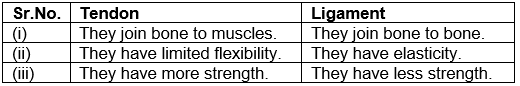(Any two)

Q.22. What do signs and symptoms indicate if a person is suffering from any disease?  (2 Mark)
Ans.
Symptoms indicate that there may be a disease, but don’t indicate what the disease is. Sign of a disease is a definite indication of the presence of a particular disease. Physicians get laboratory test done to pinpoint the disease.

Q.23. Calculate the number of moles for 56 g of Ne. (Atomic mass Ne = 20 u)  (2 Mark)
Ans. No. of moles = m/M = Given mass/molar mass
= 56/20 = 2.8 moles

OR
Differentiate between : (i) Atom and molecule (ii) Atomic number and Mass number  (2 Mark)
Ans. (i) Atoms cannot exist independently whereas molecules can exist independently
(ii) Atomic number: Total number of protons present in the nucleus of an atom.
Mass number: Sum of the total number of protons and neutrons present in the nucleus of an atom.

Q.24. What is cytoplasm? State its function.  (2 Mark)
Ans. Cytoplasm is the fluid content of the cell, occurring between nucleus and plasma membrane. It stores several vital chemicals and is the site of certain important metabolic pathways.

Q.25. (i) What are anions?
(ii) Write the chemical formulae of the following compounds:
(a) Ammonium hydroxide (b) Calcium chloride   (2 Mark)
Ans. (i) A negatively charged ion (that gains electrons) is called an anion. e.g. Cl–, Br–
(ii) (a) NH4OH
(b) CaCl2

Q.26. Classify the following as a chemical or physical change:
(i)
Water boils to form steam.
(ii) Burning of paper.  (2 Mark)
Ans. (i) Water boils to form steam: Physical change.
(ii) Burning of paper: Chemical change.

Section - C

Q.27. What type of motion is represented by each of the following graphs?  (3 Mark)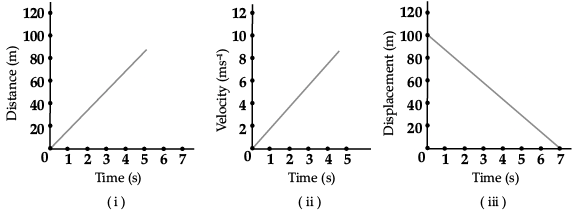Ans. (i) Uniform motion
(ii) Uniform accelerated motion
(iii) Uniform motion

OR
Which law of motion can best describe the following:

(i) Force applied while pulling a lawn mower.
(ii) Shoulder bone fracture from recoil of a gun.
(iii) Coin remains on the table, when table cloth is suddenly removed.  (3 Mark)
Ans. (i) Uniform motion
(ii) Uniform accelerated motion
(iii) Uniform motion

Q.28. Why is atomic mass of chlorine taken as 35.5 u and not a whole number like 35 u or 36 u ? Explain.  (3 Mark)
Ans. Chlorine in its natural state comprises two isotopes of atomic mass 35 u (75%) and 37 u (25%) in the ratio 3 : 1. The average atomic mass of chlorine atom is taken as:
(35 x 3/4 + 37 x 1/4) = 35.5 u which is a fixed relative atomic mass.

Q.29. State the law of conservation of energy. With the help of an example, explain the law of conservation of energy.  (3 Mark)
Ans.
Energy can neither be created nor destroyed but can be transformed from one form to another.
Explanation of law: Let us take the example of simple pendulum. We draw a pendulum bob to one side and allow it to oscillate. The pendulum bob has P. E. at the extreme positions. Then, it is converted to K.E. at the mean position and so on. It comes to rest due to air resistance and friction with the hook.
Energy is lost in overcoming friction and air resistance. But total energy remains constant.

Q.30. Define mole. How many grams of sodium will have the same number of atoms as 6 grams of magnesium? (Given Na = 23 u, Mg = 24 u)  (3 Mark)
Ans.
The mole is the amount of substance that contains the same number of particles (atoms / ions / molecules / formula units, etc.) as there are atoms in exactly 12 g of carbon-12.
Number of moles of Mg = Given mass/Molar mass = 6/24 = ¼
Number of moles of Na = ¼
Number of moles of Na = Mass of sodium / Molar mass
= 1/4 = Mass of sodium/23
Mass of sodium = 23/4 = 5.75 g
5.75 g of sodium will have the same number of atoms as 6 gm of magnesium.

Q.31. A ball is allowed to roll down from an inclined plane. It reaches the foot of the plane and continues to roll on the ground. It stops after travelling some distance. Is this the violation of law of inertia? Give reasons for your answer.  (3 Mark)
Ans. (i) No, It is not the violation of law of inertia. Law of inertia is obeyed only when no external force acts on a body. But in this case the friction due to the ground acts on the ball, so it comes to rest.
(ii) Player lowers his hand because by doing so he increases the time in which velocity of ball comes to zero. This decreases the rate of change of momentum and so the impact of force is reduced.

Q.32. (i) Write one point of difference between concentration and solubility.
(ii) What is the effect of temperature on the rate of solubility? (3 Mark)
Ans.
(i) Concentration of solution is the amount of solute present in a given amount of solution or the amount of solute dissolved in a given mass or volume of the solvent. Solubility is the maximum amount of solute that can be dissolved in a given solution at a given temperature.
(ii) The rate of solubility increases with increase in temperature.

Q.33. Give the names of the elements present in the following compounds.
(a) Quick lime
(b) Hydrogen bromide
(c) Baking powder  (3 Mark)
Ans.
(a) Calcium and oxygen
(b) Hydrogen and bromine
(c) Sodium, hydrogen, carbon and oxygen

Section - D

Q.34. (a) What is the name given to the thread shaped structures in the nucleus? Why is it important ?
(b) Draw a diagram of the nucleus to show the given parts : (i) nucleolus (ii) nuclear pore (iii) nuclear envelope  (5 Mark)

Ans. (a) The thread shaped structures in the nucleus are known as chromosomes. These are important because they contain information for inheritance of features from parents to the next generation.

(b)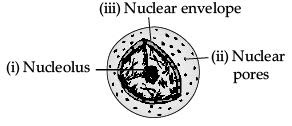OR
(a) What kind of food is advised when we fall sick and why?
(b) Mention any three basic conditions required for good health.  (5 Mark)
Ans.
(a) (i) We should take bland and nourishing food. Such food does not contain fat, oil or spices, so digested easily.
(ii) It provides sufficient energy and nutrients which are required for recovery and regeneration.

(b) (i) Balanced diet
(ii) Personal hygiene
(iii) Clean surrounding
(iv) Clean food and water
(v) Clean air
(vi) Exercises and relaxation
(vii) No addiction
(viii) Good economic condition

Q.35. Explain Rutherford’s a-particles scattering experiment with diagram and observations.   (5 Mark)
Ans. Rutherford bombarded a stream of α-particles on a thin gold foil.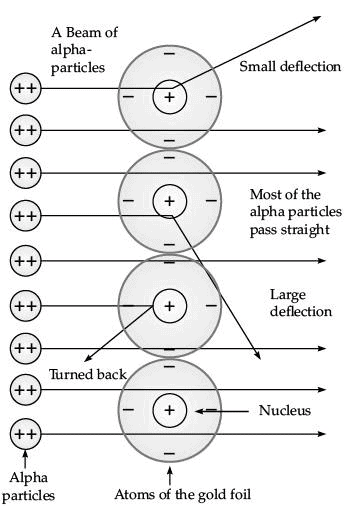(i) Most of the α-particles passed through the foil without any deflection.
(ii) A few α-particles were deflected through small angles and few through larger angles
(iii) The number of α-particles that bounced back was very small.

The important conclusions drawn from the experiment are:
(i) An atom consists of positively charged centre called nucleus.
(ii) The electrons revolve around the nucleus in well defined orbits.
(iii) The size of the nucleus is very small as compared to the size of the atom.

Q.36. (i) A sample of vitamin C is known to contain 2.58 × 1024 oxygen atoms. How many moles of oxygen atoms are present in the sample?
(ii) Write one word for the following :
(a) In a balanced chemical equation, the sum of the masses of reactants and products remains unchanged.
(b) A group of atoms carrying a fixed charge on them.
(iii) Write chemical formulae of the following compounds :
(a) Sodium phosphate
(b) Ammonium carbonate   (5 Mark)

Ans. (i) Number of moles = Given no. of particles / Avogadro number
= 2.58 x 1024 / 6.022 x 1023
= 0.4284 × 10
= 4.284 moles.
(ii) (a) Law of conservation of mass.
(b) Ion
(iii) (a) Na3PO4
(b) (NH4)2CO3

OR
(i) State the law of conservation of mass. In a chemical reaction 4.2 g of sodium hydrogen carbonate reacted with 3.0 g of ethanoic acid. The products obtained were 4.1 g of sodium ethanoate, 0.9 g of water and 2.2 g of carbon dioxide. Show that these observations are in agreement with the law of conservation of mass.
(ii) Define molecular mass. Find the relative molecular mass of calcium chloride. (Given Ca = 40 u ; Cl = 35.5 u)   (5 Mark)

Ans. (i) Mass can neither be created nor destroyed in a chemical reaction.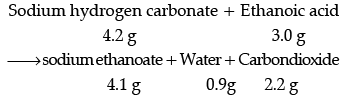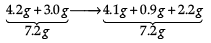These observations are in agreement with the law of conservation of mass because in a chemical reaction total mass of reactants is equal to total mass of the products.
(ii) The molecular mass of a substance is the sum of the atomic masses of all the atoms in a molecule of the substance.
Molecular mass of calcium chloride
CaCl2 = 40 + 35.5 × 2 = 111.0 u

Offer running on EduRev: Apply code STAYHOME200 to get INR 200 off on our premium plan EduRev Infinity!

135 docs

,

,

,

,

,

,

,

,

,

,

,

,

,

,

,

,

,

,

,

,

,

;